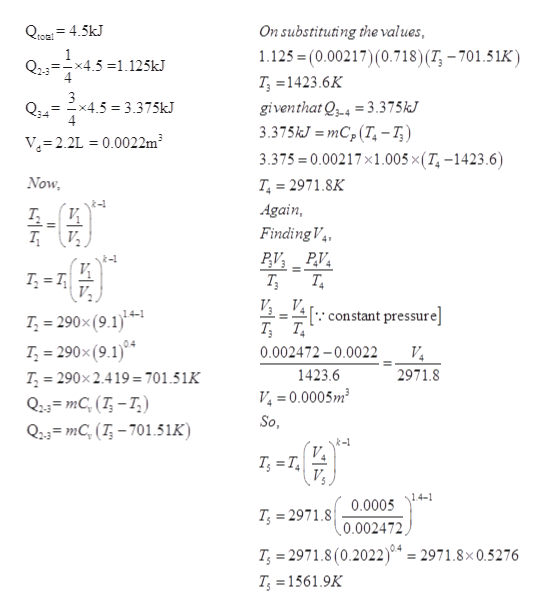# An air-standard dual cycle has a compression ratio of 9.1 and displacement of Vd = 2.2 L. At the beginning of compression, p1 = 95 kPa, and T1 = 290 K. The heat addition is 4.5 kJ, with one quarter added at constant volume and the rest added at constant pressure. Determine:a)    each of the unknown temperatures at the various states, in K.b)    the net work of the cycle, in kJ.c)    the power developed at 3000 cycles per minute, in kW.d)    the thermal efficiency.e)    the mean effective pressure, in kPa.

Question
202 views
An air-standard dual cycle has a compression ratio of 9.1 and displacement of Vd = 2.2 L. At the beginning of compression, p95 kPa, and T1 = 290 K. The heat addition is 4.5 kJ, with one quarter added at constant volume and the rest added at constant pressure. Determine:

a)    each of the unknown temperatures at the various states, in K.
b)    the net work of the cycle, in kJ.
c)    the power developed at 3000 cycles per minute, in kW.
d)    the thermal efficiency.
e)    the mean effective pressure, in kPa.
check_circle

Step 1

Part(a).

Given:

Compression ratio = 9.1

Vd = 2.2L

P1 = 95kPa

T1 = 290K

Qtotal = 4.5kJ

Step 2

Solution:

T1=290K

T2=701.51K

T3=1423.6K

T4=2971.8K

T5=1561.9Khelp_outlineImage TranscriptioncloseQIca45k On substituting the values, - toat 1.125(0.00217) (0.718) (7 - 701.51K) 4.5 1.125k 4 T 1423.6K giventhat Q3.375KJ 3.375kJ mC (T-T;) 3.375 0.00217x1.005 x(7-1423.6) 3 x4.5 3.375kJ 4 Q34= 2.2L 0.0022m3 Now T 2971.8K Again Finding PV_PV T3 4 m T T V. =4:constant pressure T T T 290x (9.1) T290x (9.1) T 290x 2.419= 701.51K 14-1 0.002472-0.0022 2971.8 1423.6 V = 0.0005m2 So QmC, (7-7) Q3s= mC, (T-701.51K) k-1 4 T T V 1.4-1 0.0005 T 2971.8 0.002472 T 2971.8(0.2022)* = 2971.8x0.5276 T 1561.9K fullscreen
Step 3

Part (b):

Net work of the c...

### Want to see the full answer?

See Solution

#### Want to see this answer and more?

Solutions are written by subject experts who are available 24/7. Questions are typically answered within 1 hour.*

See Solution
*Response times may vary by subject and question.
Tagged in